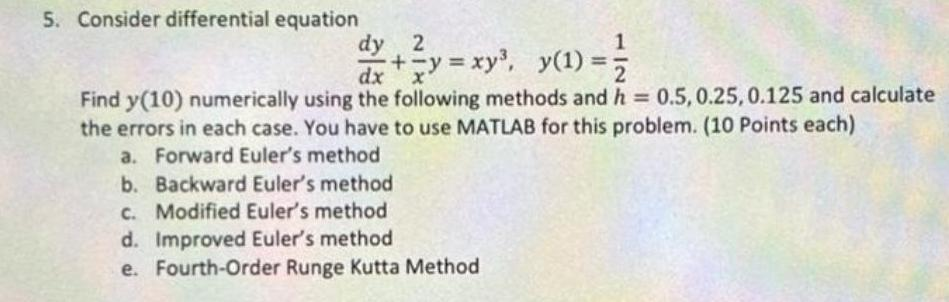Question:

# 5. Consider differential equation dy/dx + (2/x) y = xy³,

Last updated: 8/14/20225. Consider differential equation dy/dx + (2/x) y = xy³, y(1) = 1/2 Find y(10) numerically using the following methods and h = 0.5, 0.25, 0.125 and calculate the errors in each case. You have to use MATLAB for this problem. (10 Points each) a. Forward Euler's method b. Backward Euler's method c. Modified Euler's method d. Improved Euler's method e. Fourth-Order Runge Kutta Method